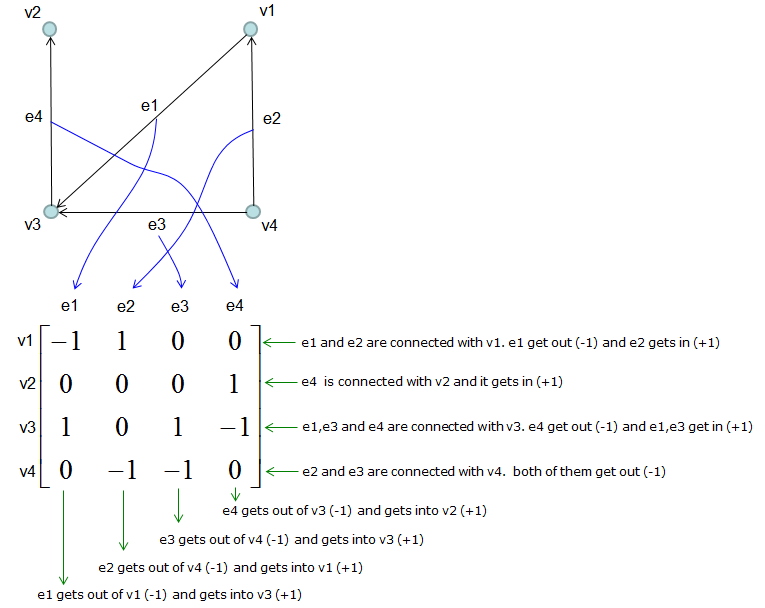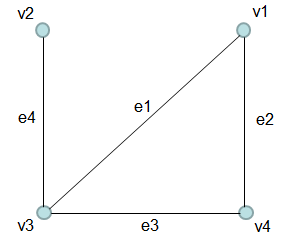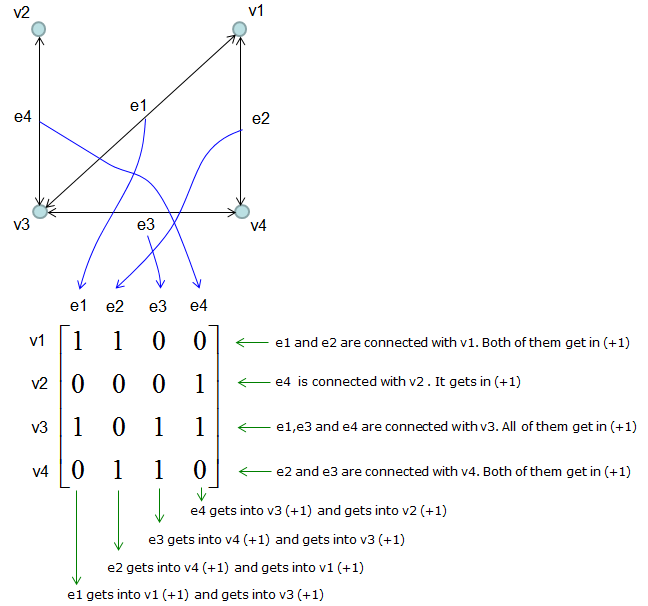Incidence Matrix Incidence Matrix is a type matrices that represents the connections between vertices and edges in a graph. The meaning of each columns and rows of the matrix can be illustrated as follows.   Case 1 : Directed GraphCase 2 : Non-Directed Graph   This is the case for Non-Directed graph. An example is as follows.Since the sign of the number in incidence matrix is determined by the direction of the edge. You may be a little confused on how to set the sign of the number in case of Non-directed Graph. There is a simple solution for the confusion.  You can think of the non-directed graph as a directed graph but all of the egdes have arrow heads on both ends. Once you convert the graph like this, you can easily construct the incidence matrix as you did in the case of directed graph as shown below.